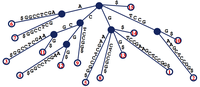# Encoding Suffix Trees solved by 395

Aug. 23, 2012, midnight by btjaden

## Creating a Suffix Tree

In “Finding the Longest Multiple Repeat”, we introduced the suffix tree. This data structure has a wide array of applications, one of which was to help us identify long repeats in a genome. In that problem, we provided the tree as part of the dataset, but a vital computational exercise is to create the suffix tree solely from a string.

## ProblemFigure 1. The suffix tree for s = GTCCGAAGCTCCGG. Note that the dollar sign has been appended to a substring of the tree to mark the end of s. Every path from the root to a leaf corresponds to a unique suffix of GTCCGAAGCTCCGG, and each leaf is labeled with the location in s of the suffix ending at that leaf.

Given a string $s$ having length $n$, recall that its suffix tree $T(s)$ is defined by the following properties:

• $T(s)$ is a rooted tree having exactly $n$ leaves.


## Sample Output

AAATG$G$
T
ATG$TG$
A
A
AAATG$G$
T
G